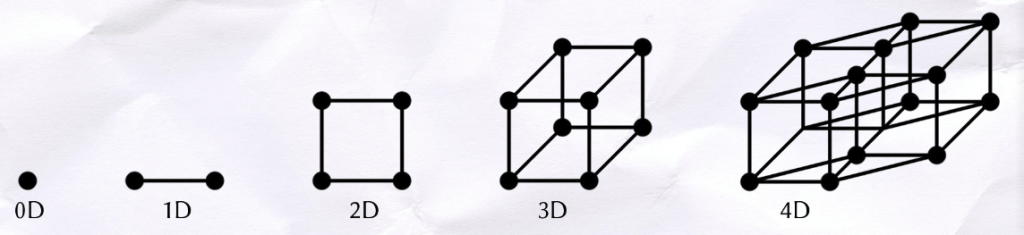# Origami tesseract

Folding origami, building networks, making projections and multiple dimensions!Like Matt Parker’s drinking straws held together by pipe cleaners, this is an easy way to explore the symmetry of the
four-dimensional companion of the cube without fancy modelling software or a rapid prototyping machine. The wireframe oblique projection uses 24 sheets of A4 paper to create two classic origami cubes placed in parallel and joins their vertices with slanted beams. In a real tesseract these connections are made through a fourth axis but unfortunately I didn’t have the time to rip a hole into another dimension. Nevertheless, the model is a simple way in which to begin to understand the shape as a continuation of the dimensions we already experience. A point is to a line as a line is to a square, a square is to a cube as a cube is to a cubic prism.The image on the right, which appears frequently in pop culture, is the perspective projection. The cube is projected away into 4D space and diminishes in volume just as the rear face of a cube appears to have a smaller area. However, the oblique projection remains my favourite since it somewhat resembles a rhombic dodecahedron, which is also the vertex-first projection of this hypercube. In other words, it is what we would see if one were to fall corner-first through our three dimensional ‘plane’. This particular way of falling exposes the maximum volume, just as a particular plane slice of a cube can expose a maximum area. Coincidentally, the number of vertices of the rhombic dodecahedron at a distance of 0, 1, 2, 3, 4 from any given vertex respectively, are 1, 4, 6, 4, 1—just as in the fourth row of Pascal’s triangle.

Schläfli symbols used by mathematicians are a compact, recursive description of regular polytopes and tessellations. In two dimensions, a triangle is simply $\{3\}$, a square is $\{4\}$ and all regular $p$-sided polygons are $\{p\}$. In three dimensions, polyhedra with $q$ regular $p$-sided polygons around each vertex are represented as $\{p,q\}$; so cubes can be described as $\{4,3\}$, for example. A tesseract, $\{4,3,3\}$, has 3 cubes around an edge. The Dali cross, Corpus Hypercubus, a net of the hypercube, uses eight cube cells, giving rise to the 8-cell as another name for the shape. Just as in the net of a cube, the tesseract’s faces must be lifted one dimension higher in order to fit them together. Once constructed, each vertex of the tesseract has four edges, giving it the vertex figure of a regular tetrahedron. With the corners removed to expose new faces you would be left with its dual polytope, a 16-cell $\{3,3,4\}$.

Hypercubes sound like the avant-garde invention of a mad mathemagician, but their geometry is being used in parallel computing. Processor networks like IBM’s Blue Gene are able to execute many calculations simultaneously from the bit to the task level, reaching a number of floating point operations per second of the 15th order of magnitude. Hypercube networks also have the advantage of being decentralised, meaning that there is no single node which could cause them to fail. A hypercube of $N$ dimensions has $2^N$ vertices, each of degree $N$. The nodes are conventionally labelled in binary with $N$ digits, where directly adjacent nodes differ by one binary digit. The longest path between two nodes in such a network is of length $N$.

You can find out more about shapes in higher dimensions from the Numberphile channel on YouTube, the MathWorld website and Matt Parker’s book Things to Make and Do in the Fourth Dimension.Lulu is an A-level student and a wannabe mathemagician
+ More articles by Lulu